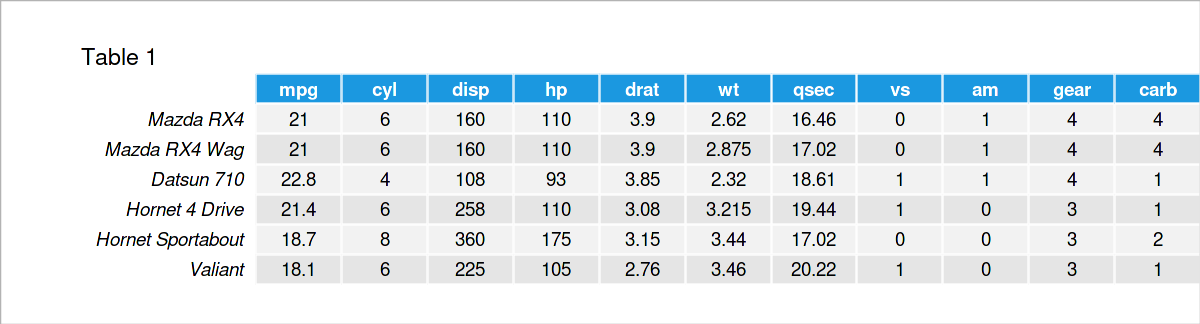# Meaning of Tilde (~) & Dot (.) in Model Formula in R (2 Examples)

In this article, I’ll demonstrate how to use tilde and dot in a model formula in R. To be more specific, the content of the tutorial looks as follows:

Here’s the step-by-step process!

## Exemplifying Data

The first step is to create some data that we can use in the following examples. I will use the built-in mtcars dataset of R, which shows the fuel consumption (mpg) and 10 aspects of automobile design and performance (cyl, disp, etc.) for 32 automobiles.

```data(mtcars)                             # sample dataThe output shows the top six rows of our example data and that our data has eleven columns. Next, we will use this dataset’s variables to set our statistical models.

## Example 1: Use of Tilde in R Models

The function of the tilde operator `~` is to separate the independent and dependent variables in a model. Let’s build a linear regression model using the mpg variable as the dependent variable and the wt variable as the independent one.

```model1 <- lm(mpg ~ wt, data = mtcars)    # model using tilde

summary(model1)                          # summary of model1
# Call:
#   lm(formula = mpg ~ wt, data = mtcars)
#
# Residuals:
#   Min      1Q  Median      3Q     Max
# -4.5432 -2.3647 -0.1252  1.4096  6.8727
#
# Coefficients:
#              Estimate Std. Error t value Pr(>|t|)
# (Intercept)  37.2851     1.8776  19.858  < 2e-16 ***
#   wt         -5.3445     0.5591  -9.559  1.29e-10 ***
#   ---
# Signif. codes:  0 ‘***’ 0.001 ‘**’ 0.01 ‘*’ 0.05 ‘.’ 0.1 ‘ ’ 1
#
# Residual standard error: 3.046 on 30 degrees of freedom
# Multiple R-squared:  0.7528,	Adjusted R-squared:  0.7446
# F-statistic: 91.38 on 1 and 30 DF,  p-value: 1.294e-10```

You can see that the model is successfully conducted, and the variables are treated as desired. In other words, the weight (wt) ‘s effect on the fuel consumption (mpg) is estimated. See the value `-5.3445` above.

## Example 2: Use of Dot in R Models

As early said, tilde `~` is used to indicate the independent and dependent variables. In cases where we want to estimate all possible main effects, we can simply use dot `.` on the right-hand side of tilde `~`. It is a useful shortcut for writing all effects in the formula. Let’s see the implementation below!

```model2 <- lm(mpg ~ ., data = mtcars)     # model using dot

summary(model2)                          # summary of model2
# Call:
# lm(formula = mpg ~ ., data = mtcars)
#
# Residuals:
#     Min      1Q  Median      3Q     Max
# -3.4506 -1.6044 -0.1196  1.2193  4.6271
#
# Coefficients:
#             Estimate Std. Error t value Pr(>|t|)
# (Intercept) 12.30337   18.71788   0.657   0.5181
# cyl         -0.11144    1.04502  -0.107   0.9161
# disp         0.01334    0.01786   0.747   0.4635
# hp          -0.02148    0.02177  -0.987   0.3350
# drat         0.78711    1.63537   0.481   0.6353
# wt          -3.71530    1.89441  -1.961   0.0633 .
# qsec         0.82104    0.73084   1.123   0.2739
# vs           0.31776    2.10451   0.151   0.8814
# am           2.52023    2.05665   1.225   0.2340
# gear         0.65541    1.49326   0.439   0.6652
# carb        -0.19942    0.82875  -0.241   0.8122
# ---
# Signif. codes:  0 ‘***’ 0.001 ‘**’ 0.01 ‘*’ 0.05 ‘.’ 0.1 ‘ ’ 1
#
# Residual standard error: 2.65 on 21 degrees of freedom
# Multiple R-squared:  0.869,	Adjusted R-squared:  0.8066
# F-statistic: 13.93 on 10 and 21 DF,  p-value: 3.793e-07```

As seen above, the main effects of all 10 variables are estimated successfully. For the shortcut of including all interaction effects, please see my tutorial: Include All Two-Way Interactions into Model in R.

## Video & Further Resources

Have a look at the following video, which I have published on my YouTube channel. In the video, I’m explaining the content of this article.

In addition to the video, you might want to read the related articles on my website. Some articles that are related to the use of tildes and dots in models can be found here.

In summary: This tutorial has explained how to include tilde and dot in a statistical model in R programming. Tell me about it in the comments section below if you have any further questions.

This page was created in collaboration with Cansu Kebabci. Have a look at Cansu’s author page to get more information about her professional background, a list of all his tutorials, as well as an overview of her other tasks on Statistics Globe.

Subscribe to the Statistics Globe Newsletter